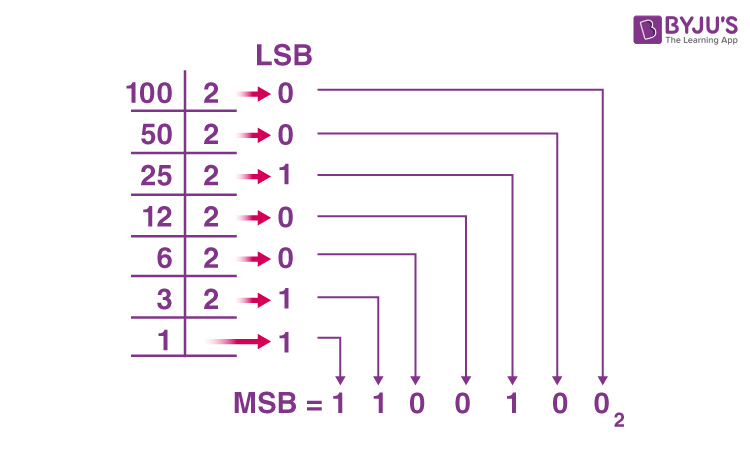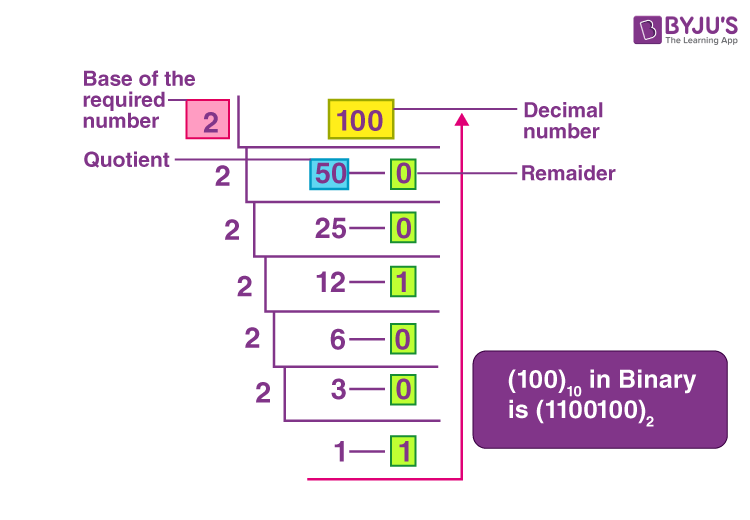# 100 in Binary

In mathematics, binary and decimal numbers are the most commonly used number systems. Binary numbers have base 2. Binary numbers are generally used in computer applications, where binary numbers are represented by only two symbols or digits, i.e. 0 (zero) and 1 (one). The binary numbers here are expressed in the base-2 numeral system. For example, (120)2 is a binary number. Each digit in this system is said to be a bit. Also, it is possible to represent the decimal number 100 in binary system.

In the decimal number system, the numbers are represented with a base 10. The way of denoting the decimal numbers with the base 10 is also known as decimal notation. It is also called the base-10 number system, which consists of 10 digits, such as 0,1,2,3,4,5,6,7,8,9. This number system is widely used in computer applications. Each digit in the decimal system has a position, and every digit is ten times more significant than the previous digit.

However, it is possible to convert numbers from decimal to binary and binary to decimal. In this article, you will learn how to convert the decimal number 100 into a binary system.

## What is 100 in Binary?

The binary equivalent of 100 is 1100100. As we know, to convert any number from the decimal system to binary, we have to divide the number by 2 and keep track of the remainder. To convert decimal to binary numbers, proceed with the steps given below:

Step 1: Divide the given decimal number by “2”, where it provides the result along with the remainder.

Step 2: If the given decimal number is even, then the result will be a whole number, and it provides the remainder with “0.”

Step 3: If the given decimal number is odd, then the result is not appropriately divided, and it provides the remainder with “1”.

Step 4: By placing all the remainders in order in such a way, the Least Significant Bit (LSB) at the top and Most Significant Bit (MSB) at the bottom, the required binary number will be obtained.

The below figure shows the conversion of the number 100 to a binary number.### 100 in Binary number

The representation of 100 in a binary number can be done as shown below.The above figure depicts the division method of converting 100 to a binary number.

## 1 to 100 in Binary

Go through the table given below to know the conversion of numbers from 1 to 100 in the binary system.

 Decimal Binary Decimal Binary 1 1 51 110011 2 10 52 110100 3 11 53 110101 4 100 54 110110 5 101 55 110111 6 110 56 111000 7 111 57 111001 8 1000 58 111010 9 1001 59 111011 10 1010 60 111100 11 1011 61 111101 12 1100 62 111110 13 1101 63 111111 14 1110 64 1000000 15 1111 65 1000001 16 10000 66 1000010 17 10001 67 1000011 18 10010 68 1000100 19 10011 69 1000101 20 10100 70 1000110 21 10101 71 1000111 22 10110 72 1001000 23 10111 73 1001001 24 11000 74 1001010 25 11001 75 1001011 26 11010 76 1001100 27 11011 77 1001101 28 11100 78 1001110 29 11101 79 1001111 30 11110 80 1010000 31 11111 81 1010001 32 100000 82 1010010 33 100001 83 1010011 34 100010 84 1010100 35 100011 85 1010101 36 100100 86 1010110 37 100101 87 1010111 38 100110 88 1011000 39 100111 89 1011001 40 101000 90 1011010 41 101001 91 1011011 42 101010 92 1011100 43 101011 93 1011101 44 101100 94 1011110 45 101101 95 1011111 46 101110 96 1100000 47 101111 97 1100001 48 110000 98 1100010 49 110001 99 1100011 50 110010 100 1100100

### What is 1 in Binary?

The decimal number 1 in the binary system is also 1. That means the binary equivalent of 1 is 1 only.

100 Billion in Binary

100 Billion = 1011101001000011101101110100000000000

### 100 in Binary to Decimal

We have converted the decimal number 100 into binary. In this section, you can learn how to convert binary to decimal, i.e. (1100100)2 to decimal. For this, we can use the formula given below:

$$\begin{array}{l} N = b_{n}q^{n} + b_{n-1}q^{n-2} + ….. + b_{2}q^{2} + b_{1}q^{1} + b_{0}q^{0} + b_{-1}q^{-1} + b_{-2}q^{-2}\end{array}$$

Where,

N is decimal equivalent,

b is the digit,

q is the base value that starts from the most significant digit order qn to least significant order q-1, q-2, …..

In other words, we need to multiply each digit of the binary number by the corresponding power of 2 and then sum up to get the required decimal value.

(1100100)2 = 1 x 26 + 1 x 25 + 0 x 24 + 0 x 23 + 1 x 22 + 0 x 21 + 0 x 20

= 64 + 32 + 0 + 0 + 4 + 0 + 0

= 100

Therefore, (1100100)2 = (100)10

To get more information about conversion of numbers from one number system to another, visit byjus.com today and get solved examples of the same for better understanding.

## Frequently Asked Questions – FAQs

Q1

### What is the binary of 100?

The binary equivalent of 100 is 1100100.

Q2

### What number is 101 in binary?

In the binary number system, 100 can be represented as 1100101.

Q3

### What number is 110 in binary?

The decimal number 110 can be represented in the binary system as 1101110.

Q4

### How do you represent 200 in binary?

We can represent 200 in binary as 11001000.

Q5

### How do you write 500 in binary?

We can write 500 in binary as 111110100.

Q6

### What is the binary for 255?

The binary equivalent of the decimal number 255 is 11111111.